# Seven Brain Twisters Using Coins & Matchsticks

Knoji reviews products and up-and-coming brands we think you'll love. In certain cases, we may receive a commission from brands mentioned in our guides. Learn more.
Challenge your mind with the following seven brain twisters: Four-square problem, rearrange the coins, the great chain puzzle, back to front, don't touch, and shift. You need only coins and matchsticks to twist your great brain.

Challenge your mind with the following Brain Twisters. You need only coins and matchsticks to exercise your right and left brain.

Four-square

Problem: Set up, with matches, the five-square formation that you see below. Then, by moving just two of the matches, form four squares — each touching another — instead of five.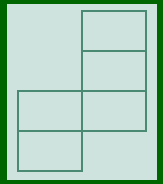Rearrange the Coins

Six coins are arranged like in the figure below.  Problem: move just one coin to another so that two rows, containing four coins each when added up either horizontally or vertically, will be formed.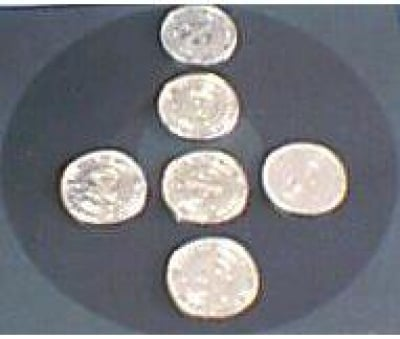The Great Chain Puzzle

If it costs a dollar to break a link, and two dollars to weld it again, what is the least it would cost to join in a single length of chain the five segments shown here?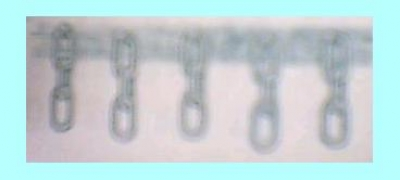Twelve dollars? Try again. The correct answer is less. There’s a simple moral lesson to this. The obvious way is not always the most economical way.

Back to Front

A triangle of 10 coins is pointed away from you. Moving only three coins, make the triangle point toward you.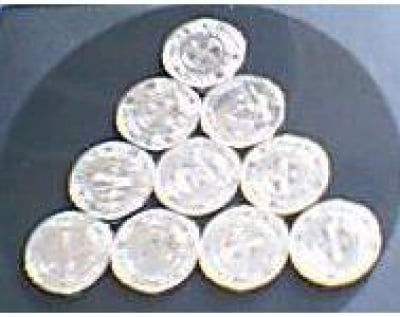Don’t Touch

Arrange 10 match sticks so that they create the equation in Roman numerals: XI + I = X. This equation is, of course, incorrect. Make the equation read correctly without touching anything.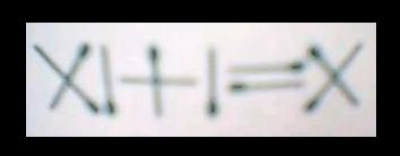Foursquare

Arrange four identical rectangular cards in the positions shown. Now form a square by moving only one card.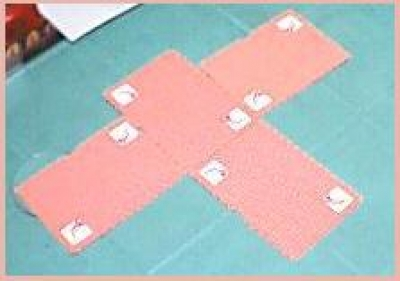Shift

Set a 10 cents coin between two one dollar coins with its edge touching both. Now get the right-hand dollar coin into the middle position — without moving 10 cents coin or touching the left-hand dollar coin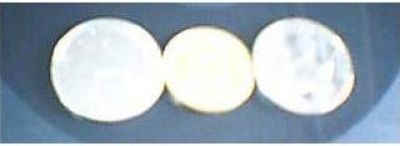Solutions:

1. Four-square problem. See figure below.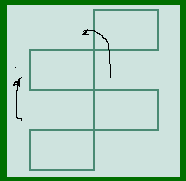2. Rearrange the coins. Move the top coin down and place it on top of the center coin. Now the coins add up to four, both horizontally and vertically.  See figure below.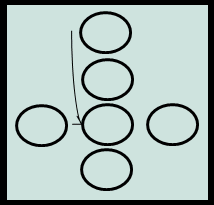3. The Great Chain Puzzle. The answer is nine dollars. Take one of the groups of three links and break each one (cost, three dollars). Then use each link to join two of the other groups (cost of three welding jobs, six dollars).

4. Move 7 to left of 2; 10 to right of 3; then 1 below and between 8 and 9.

5. Go round to the other side of the table and look.

6. Move the top card upwards until the opened space forms a square.  See figure below.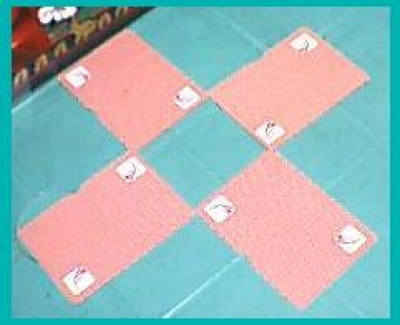7. With your left index finger, press firmly on the cents coin. With two right-hand fingers, slide the right-hand dollar coin to the right, then strike it firmly against the ten cents coin. The left-hand dollar coin will spring aside. Move the right-hand dollar coin into the exposed space.

Source: The Magic Number and seven other brain twisters brochure

## 1 comment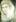lucia anna
0
Posted on Jan 28, 2011